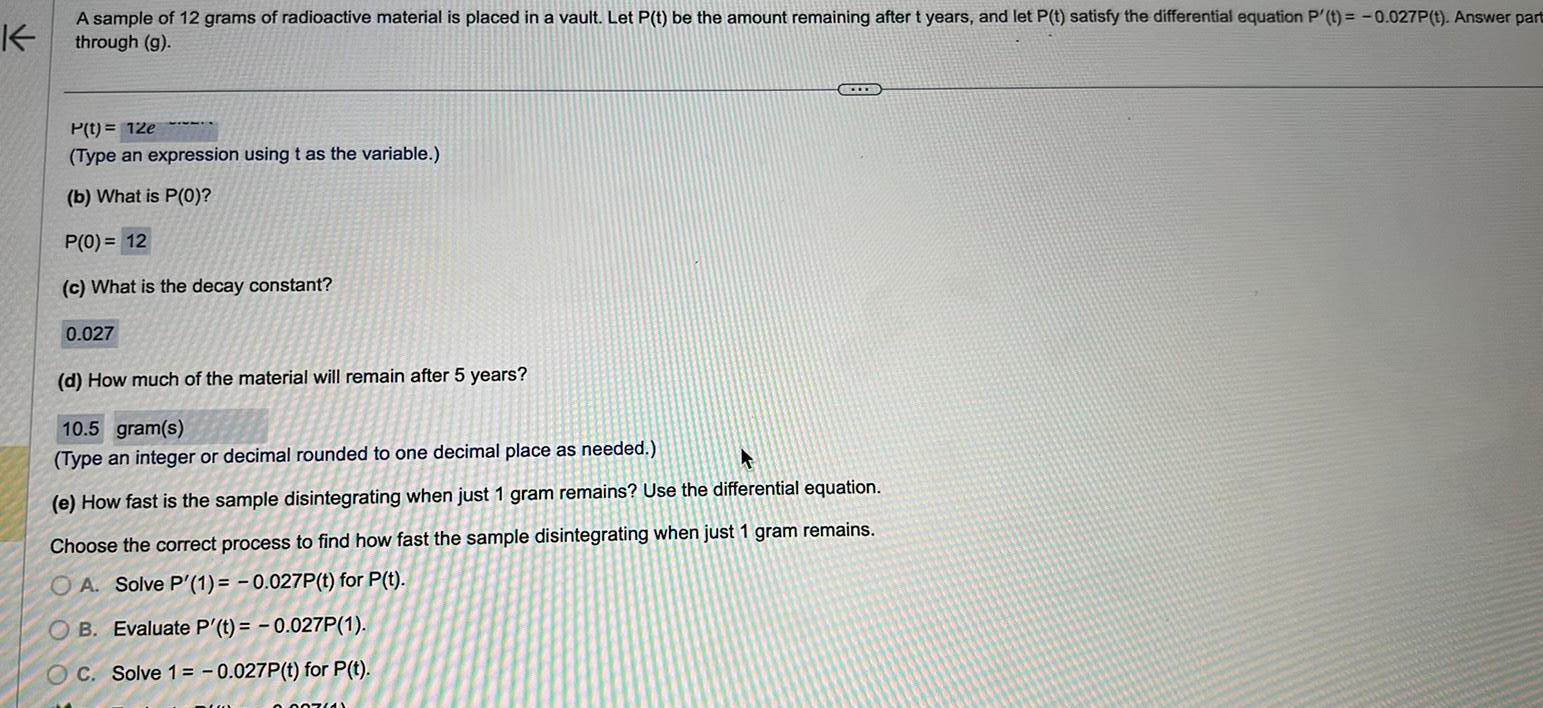Question:

# K A sample of 12 grams of radioactive material is placed in

Last updated: 9/19/2023K A sample of 12 grams of radioactive material is placed in a vault Let P t be the amount remaining after t years and let P t satisfy the differential equation P t 0 027P t Answer part through g P t 12e Type an expression using t as the variable b What is P 0 P 0 12 c What is the decay constant 0 027 d How much of the material will remain after 5 years 10 5 gram s Type an integer or decimal rounded to one decimal place as needed A e How fast is the sample disintegrating when just 1 gram remains Use the differential equation Choose the correct process to find how fast the sample disintegrating when just 1 gram remains OA Solve P 1 0 027P t for P t OB Evaluate P t 0 027P 1 OC Solve 1 0 027P t for P t